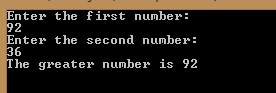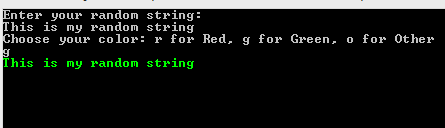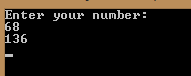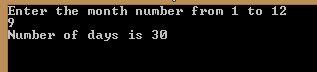In this article, we are going to talk about condition statements in C#. We will learn how to write simple condition statements, nested condition statements, and multiple condition statements.

For the complete navigation of this series check out: C# Back to Basics.

If you want to download the source code for our examples, you can do that from here Conditions in C# Source Code.

Let’s begin.

## Basic Condition Statements

If we want to execute some expression but only if some condition is met, then we need to use conditional statements. To create such a statement we need to use `if` and `else` keywords.

We can create a conditional statement like this:

```if (condition)
{
< expression1 > ;
}
else
{
< expression2 > ;
}
```

The condition is a logical expression which can result in true or false. If it is true then the `<expression1>` will be executed, otherwise, `<expression2>` will be executed. After every expression, we need to place the `;` sign.

We can execute more expressions if the condition is true or false:

```if (condition)
{
< expression1 > ;
< expression2 > ;
}
else
{
< expression3 > ;
< expression4 > ;
}
```

Example 1: Create an application which determines the greater number of two integer inputs:
```class Program
{
static void Main(string[] args)
{
Console.WriteLine("Enter the first number: ");

Console.WriteLine("Enter the second number: ");

if(first > second)
{
Console.WriteLine(\$"The greater number is {first}");
}
else
{
Console.WriteLine(\$"The greater number is {second}");
}

}
}
```We don’t have to use only `if` and `else` keywords in conditional statements, we can add another condition by adding `else if` block part:

```if(condition1)
{
< expression 1 > ;
}
else if(condition 2)
{
< expression 2 > ;
}
else if(condition n)
{
<expression n>
}
else
{
< expression k > ;
}
```

Example 2: Create an application which takes any string and the font color (r for red, g for green, o for other) as inputs. Then it needs to print out that string with the selected color:
```class Program
{
static void Main(string[] args)
{

Console.WriteLine("Choose your color: r for Red, g for Green, o for Other");

if(color == 'r')
{
Console.ForegroundColor = ConsoleColor.Red;
Console.WriteLine(sentence);
}
else if(color == 'g')
{
Console.ForegroundColor = ConsoleColor.Green;
Console.WriteLine(sentence);
}
else
{
Console.ForegroundColor = ConsoleColor.Blue;
Console.WriteLine(sentence);
}

}
}
```## Nested Conditional Statements

In C#, we can write a conditional statement inside a conditional statement if that’s one of the requirements of our project. So, the base syntax looks like this:

```if (condition)
{
if (condition2)
{
< expression1 > ;
}
else
{
< expression2 > ;
}
}
else
{
< expression3 > ;
}
```

Even though we can create nested conditional statements, we do not recommend them that much, because it would lead to low readability.

Example 3: Create an application in which the user enters a number between 1 and 100. If the number is lower then 50, our application will output multiplication by 5. But if a number is greater then 50 then for even number application will output multiplication by 2 and for an odd number application will output multiplication by 3:

```class Program
{
static void Main(string[] args)
{

if (number > 50)
{
if(number % 2 == 0) //reminder in division with two for even numbers is always a zero.
{
Console.WriteLine(number * 2);
}
else
{
Console.WriteLine(number * 3);
}
}
else
{
Console.WriteLine(number * 5);
}

}
}
```## Switch-Case Statements

In a situation where we need more than one or two conditions to execute some expression, using multiple branching could be an advantage. To use multiple branching in C#, we need to use `switch` and `case` keywords:

```switch (expression)
{
case value1:
<expression 1> ;
break;
case value2:
<expression 2> ;
break;
default:
< expression3>;
break;
}
```

Example 4: Create an application which accepts month number as an input and prints out the number of days in that month:
```static void Main(string[] args)
{
Console.WriteLine("Enter the month number from 1 to 12");

switch (month)
{
case 1: case 3: case 5:
case 7: case 8:
case 10: case 12:
Console.WriteLine("Number of days is 31");
break;
case 4: case 6:
case 9: case 11:
Console.WriteLine("Number of days is 30");
break;
case 2:
Console.WriteLine("Number of days is 28 or 29");
break;
default:
Console.WriteLine("Your number is not between 1 and 12");
break;
}